# Windows Calculator Features You Probably Don’t Know

Windows Calculator is really powerful app to calculate some useful things like dates difference, Unit conversion (angle, area, length, time, weight and more), MPG (miles per gallon), decimal / binary conversions and much more. Most of the users usually use the built-in Windows calculator for simple math calculations and don’t know that Windows calculator is packed with some amazing features. Do you want to know how many days passed since your wedding day? Would you like to know the differences between two dates? What will be the date after 37 days? What is my car consumption MPG? and lot more about unit conversions. So let’s see how we can do all these calculations.

Run Calculator, press Win+R keys from keyboard, type CALC in the Run window and hit Enter.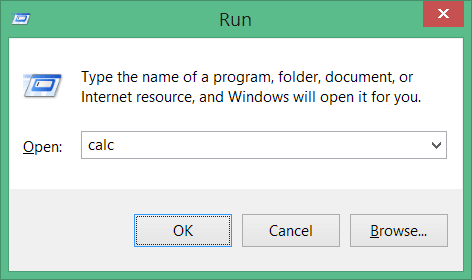## Calculate difference between two dates

Using this Date calculation feature you can either “calculate the difference between two dates” or “Add or subtract days to a specified date“.

Click the View menu and select Date Calculation option.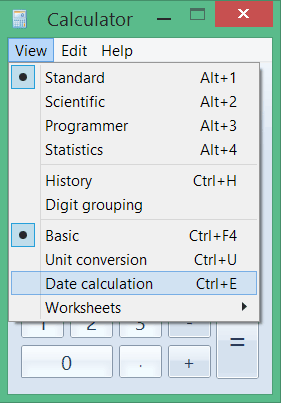As an example let’s find out the days, weeks, months and years passed since your wedding or birthday and also check the up coming date after 37 days.

• First select the difference between two dates option from drop-down menu.
• Select the From and To dates then finally hit the Calculate button, you will see the results as shown below.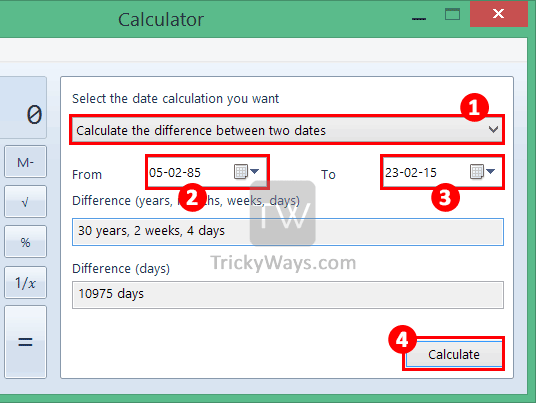## Calculate Date after number of days

In this example we are going to calculate and find a date after number of days.

• Select From date from calendar.
• Choose Add or Subtract option, in this example we will choose Add.
• Finally enter the number of day(s), month(s) or year(s) that you wan to Add or Subtract and hit the Calculate button. Results will be available in Date text box.

We have calculated the date after 37 days from today and the result is Wednesday, April 1, 2015.## Unit conversion with Windows Calculator

Unit conversion is really easy without installing extra app in Windows, you can use Windows built-in Calculator app as an unit converter. Windows Calculator is capable to convert different units like Angle, Area, Energy, Length, Power, Pressure, Temperature, Time, Velocity, Volume, Weight/Mass. So, let’s try convert temperature from Degrees Celsius to Degrees fahrenheit.

Click on View menu and select Unit conversion option.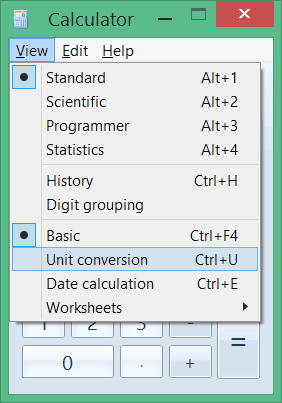• Select Temperature from unit drop-down.
• From values : Enter temperature value in Degrees Celsius and select Degrees Celsius from drop-down.
• Finally select the target temperature unit as in this example Degrees Fahrenheit.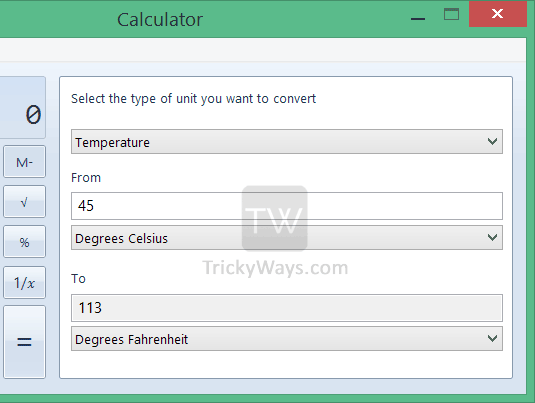## Calculate MPG (miles per gallon)

To calculate MPG using Windows Calculator, from View menu select Worksheets > Fuel economy (mpg) options.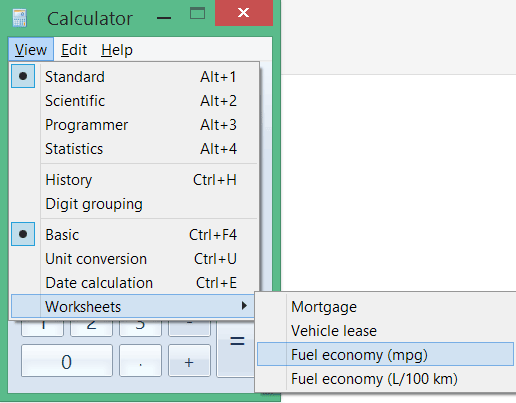Select Fuel economy (mpg) from drop-down, enter the Distance (miles) and Fuel used (gallons) values and hit the Calculate button for MPG results.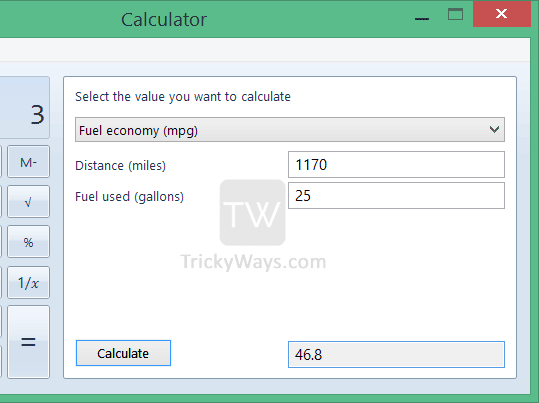## Convert Decimal to Binary

In different projects you have to covert Decimal to Binary or Binary to Decimal. In that case you can use Windows 7 or 8 built-in calculator to do it. Windows 7 and 8 calculator comes with different options like Standard, Statistics, Programmer, Scientific, Data calculation and Unit Conversion etc.

• Launch windows calculator.
• Now select “Programmer mode” from View menu or use shortcut Alt+3.
• If you want to convert Dec to Bin then first select Dec radio button, type Decimal value and then select Bin. It will convert your Decimal value into Binary. In this case I enter 25 and it will convert 25 into Binary Value 11001.• If you want to convert Bin to Dec than select Bin radio button, type your binary value and then select Dec. in this case I enter 1111 Binary value and it will convert it into Dec Value 15.

You can explorer more calculator feature that we didn’t discuss here in this guide. Some other feature to calculate Mortgage, Vehicle lease and more, go ahead and try that too.